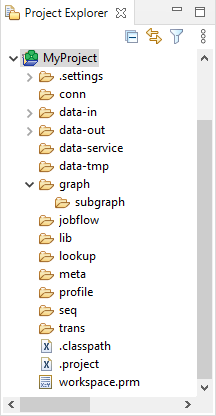#### Standard Structure of All CloverDX Projects

In the CloverDX perspective, there is a Project Explorer pane on the left side of the window. In this pane, you can expand the project folder. After that, you will be presented with the folder structure. There are subfolders for:

Table 4. Standard Folders and Parameters
Purpose Standard folder Standard parameter Parameter usage

all connections

`conn`

`CONN_DIR`

`\${CONN_DIR}`

input data

`data-in`

`DATAIN_DIR`

`\${DATAIN_DIR}`

output data

`data-out`

`DATAOUT_DIR`

`\${DATAOUT_DIR}`

temporary data

`data-tmp`

`DATATMP_DIR`

`\${DATATMP_DIR}`

graphs

`graph`

`GRAPH_DIR`

`\${GRAPH_DIR}`

subgraphs

`graph/subgraph`

`SUBGRAPH_DIR`

`\${SUBGRAPH_DIR}`

jobflows (*.jbf)

`jobflow`

`JOBFLOW_DIR`

`\${JOBFLOW_DIR}`

libraries

`lib`

`LIB_DIR`

`\${LIB_DIR}`

lookup tables

`lookup`

`LOOKUP_DIR`

`\${LOOKUP_DIR}`

`meta`

`META_DIR`

`\${META_DIR}`

profiling jobs (deprecated)

`profile`

`PROFILE_DIR`

`\${PROFILE_DIR}`

sequences

`seq`

`SEQ_DIR`

`\${SEQ_DIR}`

transformation definitions (both source files and classes)

`trans`

`TRANS_DIR`

`\${TRANS_DIR}`